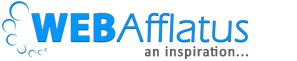# [Solved] Student Becomes the Teacher – Code Academy Problem’s SolutionHello friends! From last few days I have been learning Python and I found Code Academy extremely helpful. The way they make you to solve a big problem into small small chunk is very helpful to understand each and every steps properly and increase the problem solving skills. I have been following their tutorial on Python and recently completed one not very tough but interesting problem on the combination of List, Dictionary and Function. I hope if you came here from Google then you must be trying to find the solution of “Grading the students” problem. Though it is not advisable to look after the solution; you should try harder but if you have done all your efforts and want to get some hints here is the solution.

Problem: We need to create 3 dictionaries which have 3 students’ information like name, marks and all and then we would try to write few functions to calculate their average and class’s grades.

Solution: You can follow each steps mentioned in the tutorial and try yourself. Click here Code Academy or can refer to below code for hint.

```##########################################
## The code is written for the Code Academy tutorial.
## Author: Deepesh Singh
## Purpose: To learn and practice

##########################################
# Creating the list of 3 students with their relative information
lloyd = {
"name": "Lloyd",
"homework": [90.0, 97.0, 75.0, 92.0],
"quizzes": [88.0, 40.0, 94.0],
"tests": [75.0, 90.0]
}
alice = {
"name": "Alice",
"homework": [100.0, 92.0, 98.0, 100.0],
"quizzes": [82.0, 83.0, 91.0],
"tests": [89.0, 97.0]
}
tyler = {
"name": "Tyler",
"homework": [0.0, 87.0, 75.0, 22.0],
"quizzes": [0.0, 75.0, 78.0],
"tests": [100.0, 100.0]
}

# This function will calculate average. Say if we pass tests it will sum
# the score of tests and then divide by numbers of tests
# Example: for typer tests it would be average(tests) = (100 +100)/2 = 100
def average(numbers):
total = sum(numbers);
total = float(total)
return (total/len(numbers))

# This function will call above average function and will calculate average
# for all entities like homework, quizzes and tests
# And then will return the final result based on weightage of each entity
def get_average(student):
homework = average(student["homework"])
quizzes = average(student["quizzes"])
tests = average(student["tests"])
wt_score = (homework * .10 + quizzes * .3 + tests *.6)
print ('Average Score: %s -> %s') % (student['name'],wt_score)
return wt_score

# The function will return the final result based on the score
if score >=90:
return "A"
elif score >=80:
return "B"
elif score >= 70:
return "C"
elif score >=60:
return "D"
else:
return "F"

# In this function we will pass the list of students and will append their average score
def get_class_average(students):
#Creating a blank list
results = [];
for student in students:
#Calling average function for each student
#and then appending the result in the result list
results.append(get_average(student))
#Here we have result list which will look like result[87,78,89]
print ("\nAppened results of 3 students %s") % (results)
return average(results)

#Creating a list of all 3 students
students = [lloyd,alice,tyler]

#Calling the get_average functions for all students
final_result = get_class_average(students)
print ("\nFinal average of class:")
print final_result

```

Result:
Average Score: Lloyd -> 80.55
Average Score: Alice -> 91.15
Average Score: Tyler -> 79.9

Appened results of 3 students [80.55, 91.14999999999999, 79.9]

Final average of class:
83.8666666667